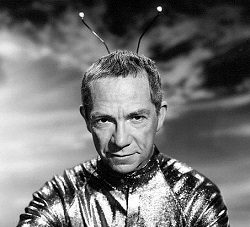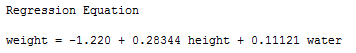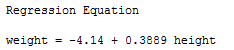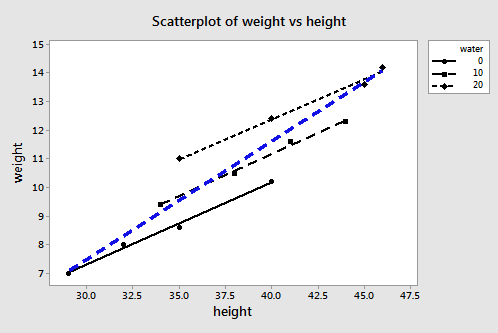# 11.1 - What if the Regression Equation Contains "Wrong" Predictors?

Before we can go off and learn about the two variable selection methods, we first need to understand the consequences of a regression equation containing the "wrong" or "inappropriate" variables. Let's do that now!

There are four possible outcomes when formulating a regression model for a set of data:

• The regression model is "correctly specified."
• The regression model is "underspecified."
• The regression model contains one or more "extraneous variables."
• The regression model is "overspecified."

Let's consider the consequence of each of these outcomes on the regression model. Before we do, we need to take a brief aside to learn what it means for an estimate to have the good characteristic of being unbiased.

### Unbiased estimates

An estimate is unbiased if the average of the values of the estimates determined from all possible random samples equals the parameter you're trying to estimate. That is, if you take a random sample from a population and calculate the mean of the sample, then take another random sample and calculate its mean, and take another random sample and calculate its mean, and so on — the average of the means from all of the samples that you have taken should equal the true population mean. If that happens, the sample mean is considered an unbiased estimate of the population mean μ.

An estimated regression coefficient bi is an unbiased estimate of the population slope βi if the mean of all of the possible estimates bi equals βi. And, the predicted response $\hat{y}_i$ is an unbiased estimate of μY if the mean of all of the possible predicted responses $\hat{y}_i$ equals μY.

So far, this has probably sounded pretty technical. Here's an easy way to think about it. If you hop on a scale every morning, you can't expect that the scale will be perfectly accurate every day —some days it might run a little high, and some days a little low. That you can probably live with. You certainly don't want the scale, however, to consistently report that you weigh five pounds more than you actually do — your scale would be biased upward. Nor do you want it to consistently report that you weigh five pounds less than you actually do — errr..., scratch that, maybe you do — in this case, your scale would be biased downward. What you do want is for the scale to be correct on average — in this case, your scale would be unbiased. And, that's what we want!

### The four possible outcomes

Now, back to the business on hand. A regression model is correctly specified (outcome 1) if the regression equation contains all of the relevant predictors, including any necessary transformations and interaction terms. That is, there are no missing, redundant or extraneous predictors in the model. Of course, this is the best possible outcome and the one we hope to achieve!

The good thing is that a correctly specified regression model yields unbiased regression coefficients and unbiased predictions of the response. And, the mean squared error (MSE) — which appears in some form in every hypothesis test we conduct or confidence interval we calculate — is an unbiased estimate of the error variance σ2.

A regression model is underspecified (outcome 2) if the regression equation is missing one or more important predictor variables. This situation is perhaps the worst-case scenario, because an underspecified model yields biased regression coefficients and biased predictions of the response. That is, in using the model, we would consistently underestimate or overestimate the population slopes and the population means. To make already bad matters even worse, the mean square error MSE tends to overestimate σ2, thereby yielding wider confidence intervals than it should.Let's take a look at an example of a model that is likely underspecified. It involves an analysis of the height and weight of martians. The data set (martian.txt) — which was obviously contrived just for the sake of this example — contains the weights (in g), heights (in cm), and amount of daily water consumption (0, 10 or 20 cups per day) of 12 martians.

If we regress y = weight on the predictors x1 = height and x2 = water, we obtain the following estimated regression equation:and the following estimate of the error variance σ2:

MSE = 0.017

If we regress y = weight on only the one predictor x1 = height, we obtain the following estimated regression equation:and the following estimate of the error variance σ2:

MSE = 0.653

Plotting the two estimated regression equations, we obtain:The three black lines represent the estimated regression equation when the amount of water consumption is taken into account — the first line for 0 cups per day, the second line for 10 cups per day, and the third line for 20 cups per day. The blue dashed line represents the estimated regression equation when we leave the amount of water consumed out of the regression model.

The second model — in which water is left out of the model — is likely an underspecified model. Now, what is the effect of leaving water consumption out of the regression model?

• The slope of the line (0.3889) obtained when height is the only predictor variable is much steeper than the slopes of the three parallel lines (0.28344) obtained by including the effect of water consumption, as well as height, on martian weight. That is, the slope likely overestimates the actual slope.
• The intercept of the line (-4.14) obtained when height is the only predictor variable is smaller than the intercepts of the three parallel lines (-1.220, -1.220 + 0.11121(10) = -0.108, and -1.220 + 0.11121(20) = 1.004) obtained by including the effect of water consumption, as well as height, on martian weight. That is, the intercept likely underestimates the actual intercepts.
• The estimate of the error variance σ2 (MSE = 0.653) obtained when height is the only predictor variable is about 38 times larger than the estimate obtained (MSE = 0.017) by including the effect of water consumption, as well as height, on martian weight. That is, MSE likely overestimates the actual error variance σ2.

This contrived example is nice in that it allows us to visualize how an underspecified model can yield biased estimates of important regression parameters. Unfortunately, in reality, we don't know the correct model. After all, if we did we wouldn't have a need to conduct the regression analysis! Because we don't know the correct form of the regression model, we have no way of knowing the exact nature of the biases.

Another possible outcome is that the regression model contains one or more extraneous variables (outcome 3). That is, the regression equation contains extraneous variables that are neither related to the response nor to any of the other predictors. It is as if we went overboard and included extra predictors in the model that we didn't need!

The good news is that such a model does yield unbiased regression coefficients, unbiased predictions of the response, and an unbiased MSE. The bad news is that — because we have more parameters in our model — MSE has fewer degrees of freedom associated with it. When this happens, our confidence intervals tend to be wider and our hypothesis tests tend to have lower power. It's not the worst thing that can happen, but it's not too great either. By including extraneous variables, we've also made our model more complicated and hard to understand than necessary.

If the regression model is overspecified (outcome 4), then the regression equation contains one or more redundant predictor variables. That is, part of the model is correct, but we have gone overboard by adding predictors that are redundant. Redundant predictors lead to problems such as inflated standard errors for the regression coefficients. (Such problems are also associated with multicollinearity, which we covered in Lesson 10).

Regression models that are overspecified yield unbiased regression coefficients, unbiased predictions of the response, and an unbiased MSE. Such a regression model can be used, with caution, for prediction of the response, but should not be used to ascribe the effect of a predictor on the response. Also, as with including extraneous variables, we've also made our model more complicated and hard to understand than necessary.

### A goal and a strategy

Okay, so now we know the consequences of having the "wrong" variables in our regression model. The challenge, of course, is that we can never really be sure which variables are "wrong" and which variables are "right." All we can do is use the statistical methods at our fingertips and our knowledge of the situation to help build our regression model.

Here's my recommended approach to building a good and useful model:

1. Know your goal, know your research question. Knowing how you plan to use your regression model can assist greatly in the model building stage. Do you have a few particular predictors of interest? If so, you should make sure your final model includes them. Are you just interested in predicting the response? If so, then multicollinearity should worry you less. Are you interested in the effects that specific predictors have on the response? If so, multicollinearity should be a serious concern. Are you just interested in summary description? What is it that you are trying to accomplish?
2. Identify all of the possible candidate predictors. This may sound easier than it actually is to accomplish. Don't worry about interactions or the appropriate functional form — such as x2 and log x — just yet. Just make sure you identify all the possible important predictors. If you don't consider them, there is no chance for them to appear in your final model.
3. Use variable selection procedures to find the middle ground between an underspecified model and a model with extraneous or redundant variables. Two possible variable selection procedures are stepwise regression and best subsets regression. We'll learn about both methods here in this lesson.
4. Fine-tune the model to get a correctly specified model. If necessary, change the functional form of the predictors and/or add interactions. Check the behavior of the residuals. If the residuals suggest problems with the model, try a different functional form of the predictors or remove some of the interaction terms. Iterate back and forth between formulating different regression models and checking the behavior of the residuals until you are satisfied with the model